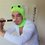# Limits.

I want to know if the following thing can be done in a limit.

i encountered this problem

$$Prove$$ $$that$$

$\large{\lim_{x\to\infty}(\dfrac{a_1^{\frac{1}{x}}+a_2^{\frac{1}{x}}+.......a_n^{\frac{1}{x}}}{n})^{nx}=a_{1}a_{2}.....a_{n}}$

$a_i\in\mathbb{R^{+}}$

$My Solution:$

We re-write $\large{a_i^{\frac{1}{x}}}$=$\large{\dfrac{a_i^{\frac{1}{x}}-1}{\frac{1}{x}}.\frac{1}{x}+1}$

doing this for all $a_i$, our expression changes to:

$\large{(\dfrac{\sum\limits_{i=1}^{n}{\dfrac{a_i^{\frac{1}{x}}-1}{\frac{1}{x}}}}{nx}+1)^{nx}}$

Now here's the part i don't think is correct:

since $x\to\infty\implies\frac{1}{x}\to 0$

for each $a_i$ : $\large{\lim_{\frac{1}{x}\to 0}\dfrac{a_i^{\frac{1}{x}}-1}{\frac{1}{x}}}=log_{e}a_{i}$.......(by standard formula)

$\therefore \large\lim_{\frac{1}{x}\to 0} \large{\sum\limits_{i=1}^{n}{\dfrac{a_i^{\frac{1}{x}}-1}{\frac{1}{x}}}=\sum\limits_{i=1}^{n}{log_{e}a_i}=log_{e}(a_{1}a_{2}....a_{n})}$

so, we have our expression as:

$\lim_{x\to\infty}\large{(1+\dfrac{log_{e}(a_{1}a_{2}....a_{n})}{nx})^{nx}}=\large{e^{log_{e}(a_{1}a_{2}....a_{n})}}=a_{1}a_{2}....a_{n}$

as required.

are the steps correct? what I mean to ask is that: can we derive the limits of the different parts independently and put their values to derive the limit of that function. 1 is this $mathematically$ $correct?$Note by Aritra Jana
6 years, 8 months ago

This discussion board is a place to discuss our Daily Challenges and the math and science related to those challenges. Explanations are more than just a solution — they should explain the steps and thinking strategies that you used to obtain the solution. Comments should further the discussion of math and science.

When posting on Brilliant:

• Use the emojis to react to an explanation, whether you're congratulating a job well done , or just really confused .
• Ask specific questions about the challenge or the steps in somebody's explanation. Well-posed questions can add a lot to the discussion, but posting "I don't understand!" doesn't help anyone.
• Try to contribute something new to the discussion, whether it is an extension, generalization or other idea related to the challenge.

MarkdownAppears as
*italics* or _italics_ italics
**bold** or __bold__ bold
- bulleted- list
• bulleted
• list
1. numbered2. list
1. numbered
2. list
Note: you must add a full line of space before and after lists for them to show up correctly
paragraph 1paragraph 2

paragraph 1

paragraph 2

[example link](https://brilliant.org)example link
> This is a quote
This is a quote
    # I indented these lines
# 4 spaces, and now they show
# up as a code block.

print "hello world"
# I indented these lines
# 4 spaces, and now they show
# up as a code block.

print "hello world"
MathAppears as
Remember to wrap math in $$ ... $$ or $ ... $ to ensure proper formatting.
2 \times 3 $2 \times 3$
2^{34} $2^{34}$
a_{i-1} $a_{i-1}$
\frac{2}{3} $\frac{2}{3}$
\sqrt{2} $\sqrt{2}$
\sum_{i=1}^3 $\sum_{i=1}^3$
\sin \theta $\sin \theta$
\boxed{123} $\boxed{123}$

Sort by:

@Parth Lohomi @Calvin Lin @Mursalin Habib @megh choksi @Satvik Golechha @HARSH SHrivastava i have just started learning about limits. so if there are any gross mistakes i apologize beforehand.

it would mean a great deal if you helped me out :D

- 6 years, 8 months ago

Sorry, er..., but I'm a -_- in calculus. Though I'm sure the calculus freak @Parth Lohomi would help.

- 6 years, 8 months ago

:D okay then

- 6 years, 8 months ago

please don't tag me everywhere!! ;(

- 6 years, 8 months ago

Sorry, thought that you'd like to aid others, howbeit thy prodigiously phenomenal and savvy calculus endowment presumably impelled me to tag you.

- 6 years, 8 months ago

:v okay this was an awesome reply :v

- 6 years, 8 months ago

oh c'mon..you cannot possibly deny that you are great at calculus!!

- 6 years, 8 months ago

@Sandeep Bhardwaj could you help? :D

- 6 years, 8 months ago

Yeah off course ! Whatever you did in your solution is absolutely correct. There is no mistake in that. There may be many different solutions to any problem with different approaches. Your approach is also good and correct. The general problems of this type $\left( 1^\infty \right)$ are done easily using the method :

Let you have to find the $L= \displaystyle Lim_{x \to a} (f(x))^{g(x)}, \quad where \quad Lim_{x \rightarrow a} f(x)=1 \quad and \quad Lim_{x \rightarrow a} g(x) =\infty$.

then, $\displaystyle L= e^{Lim_{x \rightarrow a}(f(x)-1) \times g(x)}$

You are doing great. I appreciate your this innovative approach to learn something. Very good. Keep it up. !!!

PS : The difference between a master and a beginner is that the master has failed as many times as the beginner has not even tried. ! @Aritra Jana

- 6 years, 8 months ago

thanks a ton!!! :D

You know this means a lot!!! :D

and thanks for that extra bit of info on these types of limits! is that a part of L'Hospitals rule?

- 6 years, 8 months ago

Not really L'Hospital's Rule . Because L'Hospitals Rule is applicable only for the forms of $\dfrac{0}{0}$ and $\dfrac{\infty}{\infty}$ . These limits are solved by taking the $log$ both sides.

For eg. let $y= \displaystyle Lim_{x \to a} (f(x))^{g(x)}, \quad where \quad Lim_{x \rightarrow a} f(x)=1 \quad and \quad Lim_{x \rightarrow a} g(x) =\infty$.

taking log both sides

$log(y)=g(x) \times log f(x)$

Now we solve the limit for $log(y)$..!!

And $Lim_{x \to a} g(x) \times logf(x)$ is of the form $\infty \times 0$ now, which can be further converted into $\dfrac{0}{0}$ and $\dfrac{\infty}{\infty}$ forms , and after that you can apply L'Hospitals Rule.

Suppose $lim_{x \to a} g(x) \times logf(x) = \lambda$

$\implies y= \displaystyle Lim_{x \to a} (f(x))^{g(x)}=e^\lambda$.

- 6 years, 8 months ago

ohh.. Thanks for the clarification! :D

- 6 years, 8 months ago